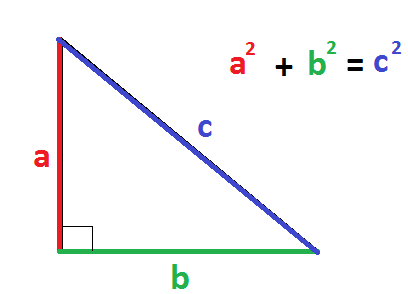# A computer system is advertised with a 14-inch monitor. The measurement of the monitor is along the diagonal of the screen. The height of the monitor screen is 9 inches. What is the width of the monitor screen?

May 19, 2018

$\sqrt{115}$ inches or about $10.724$ inches (rounded to the nearest thousandth's place).

#### Explanation:

To solve this problem we use the Pythagorean Theorem, shown here:As you can see, we can solve the missing side of a right triangle using ${\textcolor{red}{a}}^{2} + {\textcolor{g r e e n}{b}}^{2} = {\textcolor{b l u e}{c}}^{2}$ if we have the measurements of the other two sides.

In our problem, we have a diagonal (hypotenuse) of $14$ inches. We also know that the height is $9$ inches.

In the Pythagorean Theorem, that means we have $\textcolor{red}{a}$ and $\textcolor{b l u e}{c}$.

So let's plug them into the formula:
${\textcolor{red}{9}}^{2} + {\textcolor{g r e e n}{b}}^{2} = {\textcolor{b l u e}{14}}^{2}$

Simplify by squaring:
$81 + {b}^{2} = 196$

Subtract $\textcolor{\mathmr{and} a n \ge}{81}$ from both sides of the equation:
$81 + {b}^{2} \quad \textcolor{\mathmr{and} a n \ge}{- \quad 81} = 196 \quad \textcolor{\mathmr{and} a n \ge}{- \quad 81}$

${b}^{2} = 115$

Square root both sides:
$\sqrt{{b}^{2}} = \sqrt{115}$

$b = \sqrt{115}$ or about $10.724$ (rounded to nearest thousandth's place)

Therefore, the width of the monitor screen is $\sqrt{115}$ inches or about $10.724$ inches (rounded to the nearest thousandth's place).

Hope this helps!